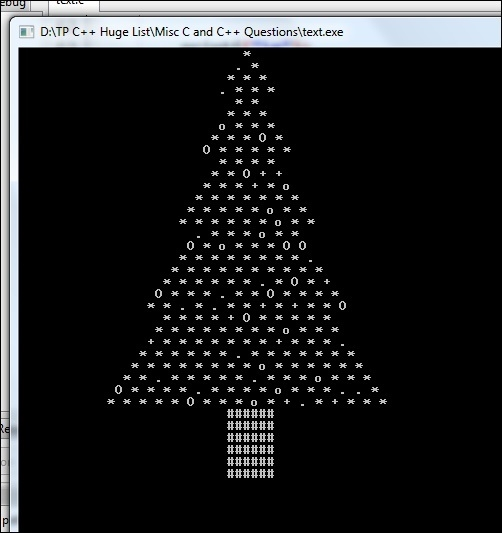# Program for Christmas Tree in C

Here we will see one interesting problem. In this problem, we will see how to print Christmas tree randomly. So the tree will be flickering like Christmas tree lights.

To print a Christmas tree, we will print pyramids of various sizes just one beneath another. For the decorative leaves a random character is printed from a given list of characters. The height and randomness is adjustable.

Here after generating a tree, the whole screen is cleared, then generate again, that’s why this is looking like flickering tree.

## Example

#include <stdio.h>
#include <stdlib.h>
#include <time.h>
#include <unistd.h>
#define REFRESH_RATE 40000
#define RANDOM_NESS 5 // The higer value indicates less random
void clear_screen() {
system("@cls||clear");
}
void display_random_leaf() {
char type_of_leaves = { '.', '*', '+', 'o', 'O' }; //these are the leaf types
int temp = rand() % RANDOM_NESS;
if (temp == 1)
printf("%c ", type_of_leaves[rand() % 5]); //if temp is 1, then use other leaves
else
printf("%c ", type_of_leaves); //otherwise print *
}
void tree_triangle(int f, int n, int toth) {
int i, j, k = 2 * toth - 2;
for (i = 0; i < f - 1; i++)
k--;
for (i = f - 1; i < n; i++) { //i will point the number of rows
for (j = 0; j < k; j++) // Used to put spaces
printf(" ");
k = k - 1;
for (j = 0; j <= i; j++)
display_random_leaf();
printf("");
}
}
void display_tree(int h) {
int start = 1, end = 0, diff = 3;
while (end < h + 1) {
end = start + diff;
tree_triangle(start, end, h);
diff++;
start = end - 2;
}
}
void display_log(int n) { //print the log of the tree
int i, j, k = 2 * n - 4;
for (i = 1; i <= 6; i++) {
for (j = 0; j < k; j++)
printf(" ");
for (j = 1; j <= 6; j++)
printf("#");
printf("");
}
}
main() {
srand(time(NULL));
int ht = 15;
while (1) {
clear_screen();
display_tree(ht);
display_log(ht);
usleep(REFRESH_RATE); //use sleep before replacing
}
}

## Output